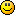# Laws of nature

Jump to Last Post 1-5 of 5 discussions (26 posts)
1.Is it possible there are laws of nature we don't know about or understand yet? How else do you explain this?2. Why do the Earth and Moon fit so perfectly into  the squared circle this way? The planet WE are on? The only planet we know of that has life?

3.And how did the builders of Stonehenge know? The numbers are even the same.

5040 miles
2160 miles
7920 miles

50.40 ft
21.60 ft
79.20 ft

1. Actually you will find a lot of numbers that relate to nature. The Fibonacci sequence for instance. Many now say mathematics is the language of nature. I tend to agree.

1. I agree. Those same numbers are found in the platonic solids, basic geometry, and lots of other places. Including the dimensions of ancient buildings.

The golden mean, is found all over nature, including what people consider to be "beautiful", like human faces, dimensions, and art. Even iphones and technology.

1. That is exactly why I love chaos theory.

4. Unfortunately, the figure you show as 5040 is actually 5029.  (7900+2157)/2

The polar diameter of earth is only 7900, not 7920 and the moon is slightly smaller as well.  When playing with numbers precision is #1.

1. I have 12,742 km as the average diameter, which is 7,926 miles. The polar is 12,713 km or 7,899 miles. Equatorial is 12,756 km.

It depends on the source you use, it seems.

1. The photo shows a polar diameter, so that's what I used (7899.98 miles).  Of course, if you moved the moon around just the right amount (not on the equatorial plane or even that of the solar system) it would work.

Of course, that's how these things often work; find a fairly close match to some unusual coincidence and start rounding and ignoring until it fits exact.

1. Agreed. I haven't investigated the picture or the claims, so I wasn't commenting on them, just the premise of the forum.

2. The universe works in ideal numbers.

Just like the ideal number for the Precession of the Equinoxes is 25,920 years (notice it's divisible by 2160) The numbers are everywhere.

1. The sources I looked gave figures of 25,772 years, 25765, 25625 and 25772.  None of which is divisible by 2160.  Precision is #1, not "adjusting" the numbers to fit mystical claims.

1. Precession can't be precisely measure, because it changes. So there is a standard set.

1. A standard figure...calculated by whom?  The people that want it to be exactly a multiple of 2160 regardless of what it actually is, or someone with the instrumentation and knowledge to measure it accurately?

Plus, of course, if it changes (and it does) then how can it be a multiple of that number?

3. Even if the numbers are wrong, the fact remains that the Earth and Moon still fit precisely into the squared circle. You can easily use ratios and see for yourself.

How do you explain it? Coincidence? I say there are laws of nature we don't know about.

1. I don't see a "squared circle" in your picture, and don't know what one is anyway.  A square with sides of Pi*2R/4 of the circle?  Your diagram says the square and circle are "nearly" the same that way, but not identical.

(By the way, why isn't the circle drawn in the proper position?  Shifting it down makes a prettier picture and gives the impression that the center of the earth is special in the diagrams, but it doesn't belong there.)

Either way, though, that 4 times the earths diameter nearly equals Pi times the sum of the earth's diameter plus the moons wouldn't seem to mean much.  Our moon is, after all, abnormally large as moons go (and the earth well under average) and that's the only reason it works at all.  I fail to see where any "laws of nature" might apply, particularly considering how the moon was formed.  I suppose that if we considered the composition, temperature and age of both, speed of collision and the angle of collision of that formation, we might find that we'd get a particular percentage of the total becoming the moon.  If there was nothing else to draw it away than what was here (other planets, sun, comets, etc.).  I wouldn't call that chance a "natural law", though.

1. A circle with the same perimeter of the square is a squared circle. They can never be exact, it's mathematically impossible.

1. Really?  Excepting that Pi is not a rational number, every circle has a "sqaured" circle.  And one much closer than what the diagram shows.  Thousands of them in fact.

To find that square, find the perimeter of the circle (Pi* diameter) and divide it by 4.  You have the square, and depending on how accurate you measure the diameter of the circle and how many decimals you carry the math to, you will have a square that is very, very close to that "squared circle".

But if you want a perfect one, use a circle with radius 2.  The perfect squared circle has a side length of Pi.  Mathematically it's easy as pie.1. I made a mistake. I meant you can't do it with a square and compass. But you can do it mathematically.

1. You lost me.  You'll need a ruler of course (to measure the compass setting as well as draw a straight line), but you can then draw both.  What am I missing?

1. I'm not good at math, so I don't know. It's just something I keep reading. It says it here.:

http://www.cut-the-knot.org/impossible/sq_circle.shtml

1. No, it gives the solution near the bottom.  My own geometry isn't good enough to follow it, but it says the solution is there.

working